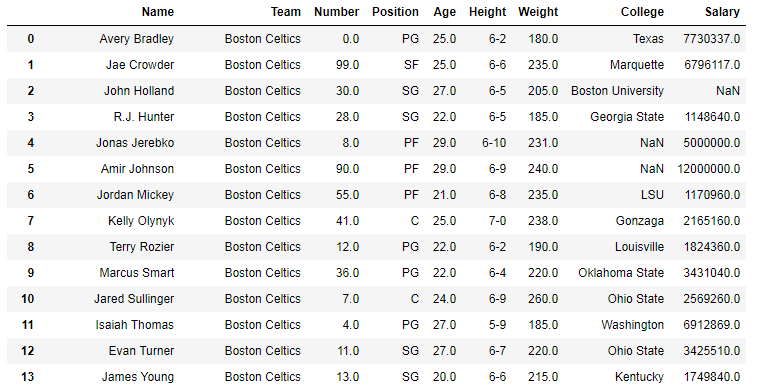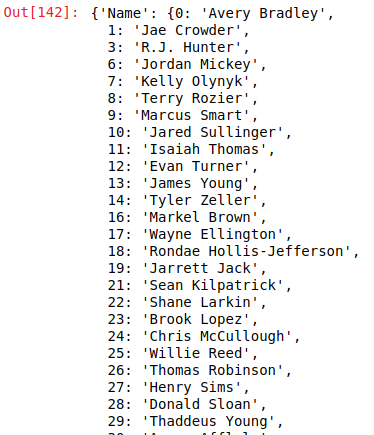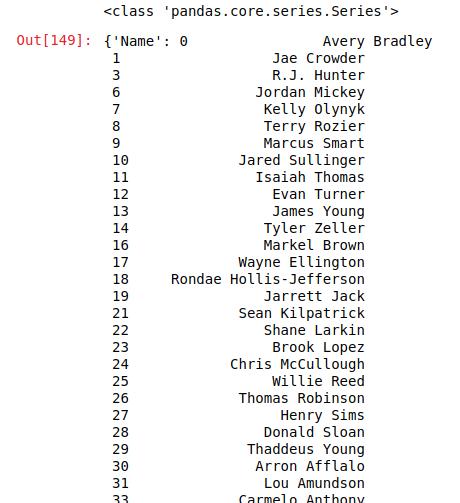# Pandas Dataframe.to_dict()

## Python | Pandas Dataframe.to_dict()\

Python is a great language for doing data analysis, primarily because of the fantastic ecosystem of data-centric Python packages. Pandas is one of those packages and makes importing and analyzing data much easier.

Pandas `.to_dict()` method is used to convert a dataframe into a dictionary of series or list like data type depending on orient parameter.

Syntax: DataFrame.to_dict(orient=’dict’, into=)

Parameters:
orient: String value, (‘dict’, ‘list’, ‘series’, ‘split’, ‘records’, ‘index’) Defines which dtype to convert Columns(series into). For example, ‘list’ would return a dictionary of lists with Key=Column name and Value=List (Converted series).
into: class, can pass an actual class or instance. For example in case of defaultdict instance of class can be passed. Default value of this parameter is dict.

Return type: Dataframe converted into Dictionary

In the following examples, the data frame used contains data of some NBA players. The image of data frame before any operations is attached below.Example #1: Default conversion into dictionary of Dictionaries
In this case, no parameter is passed to the `to_dict()` method. Hence it will convert the dataframe in to a dictionary of dictionaries by default.

 `# importing pandas module ` `import` `pandas as pd` ` ` `# reading csv file from url ` `data ``=` `pd.read_csv(``"https://media.geeksforgeeks.org/wp-content/uploads/nba.csv"``)` `  ` `# dropping null value columns to avoid errors` `data.dropna(inplace ``=` `True``)` ` ` `# converting to dict` `data_dict ``=` `data.to_dict()` ` ` `# display` `data_dict`

Output:
As shown in the output image, dictionary of dictionaries was returned by to_dict() method. The key of first dictionary is column name and the column is stored with index as key of 2nd dictionary.Example #2: Converting to dictionary of Series

In this example, ‘series’ is passed to the orient parameter to convert the data frame into Dictionary of Series.

 `# importing pandas module ` `import` `pandas as pd` ` ` `# reading csv file from url ` `data ``=` `pd.read_csv(``"https://media.geeksforgeeks.org/wp-content/uploads/nba.csv"``)` `  ` `# dropping null value columns to avoid errors` `data.dropna(inplace ``=` `True``)` ` ` `# converting to dict` `data_dict ``=` `data.to_dict(``'series'``)` ` ` `# printing datatype of first keys value in dict` `print``(``type``(data_dict[``'Name'``]))` ` ` `# display` `data_dict`

Output:
As shown in the output image, Since the type of data_dict[‘Name’] was pandas.core.series.Series, to_dict() returned a dictionary of series.Last Updated on November 13, 2021 by admin

## Sort Correlation Matrix in PythonSort Correlation Matrix in Python

Sort Correlation Matrix in Python A correlation matrix investigates the dependence between multiple variables at

## How to Do a vLookup in Python using pandasHow to Do a vLookup in Python using pandas

How to Do a vLookup in Python using pandas Vlookup is essentially used for vertically

## Align columns to Left in Pandas – PythonAlign columns to Left in Pandas – Python

Align columns to Left in Pandas – Python Pandas library is useful for performing exploratory

## Python – Pandas DataFrame.astype()Python – Pandas DataFrame.astype()

In this article, we will explore the DataFrame.astype() method in the Python pandas library. The

## Python | Pandas dataframe.infer_objects()Python | Pandas dataframe.infer_objects()

Python | Pandas dataframe.infer_objects() Python is a great language for doing data analysis, primarily because

## String manipulations in Pandas DataFrameString manipulations in Pandas DataFrame

String manipulations in Pandas DataFrame String manipulation is the process of changing, parsing, splicing, pasting, or

## Python – Split given list and insert in excel filePython – Split given list and insert in excel file

Python | Split given list and insert in excel file Given a list containing Names